# 无需 Python 或 R 语言知识的 Yandex CatBoost 机器学习算法

18 二月 2021, 08:52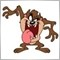0
1 242

### 预测因子

• 显著水平可以是：
• 水平的（如交易日开盘价）
• 线性的（例如，回归通道）
• 断开的（通过非线性函数计算，例如移动平均值）
• 价格和水平位置：
• 在固定点数范围内
• 在固定百分比范围内:
• 相对于当日开盘价或水平
• 相对于波动水平
• 相对于不同时间框架的趋势段
• 描述价格波动（波动性）
• 有关事件时间的信息：
• 自重要事件开始（从当前柱形图或不同时段（如当天）开始）以来经过的柱数
• 自重要事件结束后经过的柱数（从当前条或不同时段开始，如一天）
• 自事件开始和结束以来经过的柱数，显示持续时间
• 当前时间，如小时数、星期几、十年或月数、其他
• 有关事件动态的信息：
•  重要水平交叉的数量（包括考虑衰减/重复频率的计算）
•  第一次/最后一次事件发生时的最高/最低价格（相对价格）
•  事件速度（单位时间的点数）
• 将OHLC数据转换为其他坐标平面
• 振荡器类型指标的值。

### 目标

• 第一种方法: "1" — 开启一个仓位 (或者执行另一项行为) 以及 "0" — 不开启仓位 (或者不执行行为);
• 第二种方法: "1" — 开启一个买入仓位 (第一个行为) 以及 "0" — 开启一个卖出仓位 (第二个行为).

• 当买入或卖出价格水平交叉时开仓（任何指标都可以作为一个水平）；
• 根据价格相对于当日开盘价的位置，从一小时开始在第 N 个柱上开仓或忽略开仓。

### 机器学习软件

CatBoost是一个开源的机器学习算法，来自著名的Yandex公司。因此，我们可以期待相关的产品支持、改进和错误修复。

### 为机器学习准备数据

• 时间/小时数/小时分数/一周中的某一天
• 柱的相对位置
• 振荡指标

• Accelerator Oscillator（加速计振荡器）
• Average Directional Movement Index (平均方向性走势指标)
• Average Directional Movement Index by Welles Wilder (Wilder 平均方向性走势指标)
• Average True Rang (平均真实范围)e
• Bears Power (空头力度)
• Bulls Power (多头力度)
• Commodity Channel Index (商品通道指数)
• Chaikin Oscillator (Chaikin 振荡器)
• DeMarker
• Force Index (强力指数)
• Gator (鳄鱼)
• Market Facilitation Index （市场促进指数）
• Momentum （动量）
• Money Flow Index （资金流量指数）
• Moving Average of Oscillator （移动平均震荡指标）
• Moving Averages Convergence/Divergence （MACD）
• Relative Strength Index (相对强度指数)
• Relative Vigor Index (相对动量指数)
• Standard Deviation (标准方差)
• Stochastic Oscillator (随机振荡指标)
• Triple Exponential Moving Averages Oscillator (三倍指数移动平均线)
• Williams' Percent Range (威廉姆斯百分比范围)
• Variable Index Dynamic Average (可变指数动态均线）
• Volume (交易量)

• Awesome Oscillator （动量震荡器）
• On Balance Volume （平衡成交量）
• Accumulation/Distribution （离散技术指标）

• 创建表，从文件中读取表并将其保存到文件中。
• 根据单元格地址向任何表单元格读写信息。
• 表列可以存储不同的数据类型，这样可以节省内存消耗。
• 表段可以完全从指定的地址复制到另一个表的指定地址。
• 提供按任何表列筛选。
• 根据列单元格中指定的值，提供降序和升序的多级排序。
• 允许重新索引列并隐藏它们。
• 还有更多其他有用且用户友好的特性。

### EA 交易组件

1. 价格与价格的移动平均交叉。
2. 条件1满足后，价格首次未触及前一个柱上的MA。

```//+-----------------------------------------------------------------+
//| Returns a buy or Sell signal - basic strategy                   |
//+-----------------------------------------------------------------+
bool Signal()
{
// Reset position opening blocking flag
SellPrIMA=false;  // Open a pending sell order
BuyPrIMA=false;   // Open a pending buy order
SellNow=false;    // Open a market sell order
BuyNow=false;     // Open a market buy order
bool Signal=false;// Function operation result
int BarN=0;       // The number of bars on which MA is not touched
if(iOpen(Symbol(),Signal_MA_TF,0)>MA_Signal(0) && iLow(Symbol(),Signal_MA_TF,1)>MA_Signal(1))
{
for(int i=2; i<100; i++)
{
if(iLow(Symbol(),Signal_MA_TF,i)>MA_Signal(i))break;// Signal has already been processed on this cycle
if(iClose(Symbol(),Signal_MA_TF,i+1)<MA_Signal(i+1) && iClose(Symbol(),Signal_MA_TF,i)>=MA_Signal(i))
{
for(int x=i+1; x<100; x++)
{
if(iLow(Symbol(),Signal_MA_TF,x)>MA_Signal(x))break;// Signal has already been processed on this cycle
if(iHigh(Symbol(),Signal_MA_TF,x)<MA_Signal(x))
{
BarN=x;
BuyNow=true;
break;
}
}
}
}
}
if(iOpen(Symbol(),Signal_MA_TF,0)<MA_Signal(0) && iHigh(Symbol(),Signal_MA_TF,1)<MA_Signal(1))
{
for(int i=2; i<100; i++)
{
if(iHigh(Symbol(),Signal_MA_TF,i)<MA_Signal(i))break;// Signal has already been processed on this cycle
if(iClose(Symbol(),Signal_MA_TF,i+1)>MA_Signal(i+1) && iClose(Symbol(),Signal_MA_TF,i)<=MA_Signal(i))
{
for(int x=i+1; x<100; x++)
{
if(iHigh(Symbol(),Signal_MA_TF,x)<MA_Signal(x))break;// Signal has already been processed on this cycle
if(iLow(Symbol(),Signal_MA_TF,x)>MA_Signal(x))
{
BarN=x;
SellNow=true;
break;
}
}
}
}
}
if(BuyNow==true || SellNow==true)Signal=true;
return Signal;
}
```

```#include "CSV fast.mqh";                 // Class for working with tables
CSV *csv_CB=new CSV();                   // Create a table class instance, in which current predictor values will be stored

//+------------------------------------------------------------------+
//| Expert initialization function                                   |
//+------------------------------------------------------------------+
int OnInit()
{
CB_Tabl();// Creating a table with predictors
return(INIT_SUCCEEDED);
}
//+------------------------------------------------------------------+
//| Create a table with predictors                                   |
//+------------------------------------------------------------------+
void CB_Tabl()
{
//--- Columns for oscillators
Size_arr_Buf_OSC=ArraySize(arr_Buf_OSC);
Size_arr_Name_OSC=ArraySize(arr_Name_OSC);
Size_TF_OSC=ArraySize(arr_TF_OSC);
for(int n=0; n<Size_arr_Buf_OSC; n++)SummBuf_OSC=SummBuf_OSC+arr_Buf_OSC[n];
Size_OSC=3*Size_TF_OSC*SummBuf_OSC;
for(int S=0; S<3; S++)// Loop by the number of shifts
{
string Shift="0";
if(S==0)Shift="1";
if(S==1)Shift="2";
if(S==2)Shift="15";
for(int T=0; T<Size_TF_OSC; T++)// Loop by the number of timeframes
{
for(int o=0; o<Size_arr_Name_OSC; o++)// Loop by the number of indicators
{
for(int b=0; b<arr_Buf_OSC[o]; b++)// Loop by the number of indicator buffers
{
name_P=arr_Name_OSC[o]+"_B"+IntegerToString(b,0)+"_S"+Shift+"_"+arr_TF_OSC[T];
csv_CB.Add_column(dt_double,name_P);// Add a new column with a name to identify a predictor
}
}
}
}
}
//+------------------------------------------------------------------+
//--- Call predictor calculation
//+------------------------------------------------------------------+
void Pred_Calc()
{
//--- Get information from oscillator indicators
double arr_OSC[];
iOSC_Calc(arr_OSC);
for(int p=0; p<Size_OSC; p++)
{
csv_CB.Set_value(0,s(),arr_OSC[p],false);
}
}
//+------------------------------------------------------------------+
//| Get values of oscillator indicators                              |
//+------------------------------------------------------------------+
void iOSC_Calc(double &arr_OSC[])
{
ArrayResize(arr_OSC,Size_OSC);
int n=0;// Indicator handle index
int x=0;// Total number of iterations
for(int S=0; S<3; S++)// Loop by the number of shifts
{
n=0;
int Shift=0;
if(S==0)Shift=1;
if(S==1)Shift=2;
if(S==2)Shift=15;
for(int T=0; T<Size_TF_OSC; T++)// Loop by the number of timeframes
{
for(int o=0; o<Size_arr_Name_OSC; o++)// Loop by the number of indicators
{
for(int b=0; b<arr_Buf_OSC[o]; b++)// Loop by the number of indicator buffers
{
arr_OSC[x++]=iOSC(n, b,Shift);
}
n++;// Mark shift to the next indicator handle for calculation
}
}
}
}
//+------------------------------------------------------------------+
//| Get the value of the indicator buffer                            |
//+------------------------------------------------------------------+
double iOSC(int OSC, int Bufer,int index)
{
double MA= {0.0};
int handle_ind=arr_Handle[OSC];// Indicator handle
ResetLastError();
if(CopyBuffer(handle_ind,0,index,1,MA)<0)
{
PrintFormat("Failed to copy data from the OSC indicator, error code %d",GetLastError());
return(0.0);
}
return (MA);
}
```

```//+-----------------------------------------------------------------+
//| The function copies predictors to archive                       |
//+-----------------------------------------------------------------+
void Copy_Arhiv()
{
int Strok_Arhiv=csv_Arhiv.Get_lines_count();// Number of rows in the table
int Stroka_Load=0;// Starting row in the source table
int Stolb_Load=1;// Starting column in the source table
int Stroka_Save=0;// Starting row to write in the table
int Stolb_Save=1;// Starting column to write in the table
int TotalCopy_Strok=-1;// Number of rows to copy from the source. -1 copy to the last row
int TotalCopy_Stolb=-1;// Number of columns to copy from the source, if -1 copy to the last column

Stroka_Save=Strok_Arhiv;// Copy the last row
csv_Arhiv.Copy_from(csv_CB,Stroka_Load,Stolb_Load,TotalCopy_Strok,TotalCopy_Stolb,Stroka_Save,Stolb_Save,false,false,false);// Copying function

//--- Calculate the financial result and set the target label, if it is not the first market entry
int Stolb_Time=csv_Arhiv.Get_column_position("Time",false);// Find out the index of the "Time" column
int Vektor_P=0;// Specify entry direction: "+1" - buy, "-1" - sell
if(BuyNow==true)Vektor_P=1;// Buy entry
else Vektor_P=-1;// Sell entry
csv_Arhiv.Set_value(Strok_Arhiv,Stolb_Time+1,Vektor_P,false);
if(Strok_Arhiv>0)
{
int Stolb_Target_P=csv_Arhiv.Get_column_position("Target_P",false);// Find out the index of the "Time" column
int Load_Vektor_P=csv_Arhiv.Get_int(Strok_Arhiv-1,Stolb_Target_P,false);// Find out the previous operation type
datetime Load_Data_Start=StringToTime(csv_Arhiv.Get_string(Strok_Arhiv-1,Stolb_Time,false));// Read the position opening date
datetime Load_Data_Stop=StringToTime(csv_Arhiv.Get_string(Strok_Arhiv,Stolb_Time,false));// Read the position closing date
double F_Rez_Buy=0.0;// Financial result in case of a buy operation
double F_Rez_Sell=0.0;// Financial result in case of a sell operation
double P_Open=0.0;// Position open price
double P_Close=0.0;// Position close price
int Metka=0;// Label for target variable
P_Open=iOpen(Symbol(),Signal_MA_TF,iBarShift(Symbol(),Signal_MA_TF,Load_Data_Start,false));
P_Close=iOpen(Symbol(),Signal_MA_TF,iBarShift(Symbol(),Signal_MA_TF,Load_Data_Stop,false));
F_Rez_Buy=P_Close-P_Open;// Previous entry was buying
F_Rez_Sell=P_Open-P_Close;// Previous entry was selling
if((F_Rez_Buy-comission*Point()>0 && Load_Vektor_P>0) || (F_Rez_Sell-comission*Point()>0 && Load_Vektor_P<0))Metka=1;
else Metka=0;
csv_Arhiv.Set_value(Strok_Arhiv-1,Stolb_Time+2,Metka,false);// Write label to a cell
csv_Arhiv.Set_value(Strok_Arhiv-1,Stolb_Time+3,F_Rez_Buy,false);// Write the financial result of a conditional buy operation to the cell
csv_Arhiv.Set_value(Strok_Arhiv-1,Stolb_Time+4,F_Rez_Sell,false);// Write the financial result of a conditional sell operation to the cell
csv_Arhiv.Set_value(Strok_Arhiv,Stolb_Time+2,-1,false);// Add a negative label to the labels to control labels
}
}
```

```//+-----------------------------------------------------------------+
//| The function applies predictors in the CatBoost model           |
//+-----------------------------------------------------------------+
void Model_CB()
{
CB_Siganl=1;
csv_CB.Get_array_from_row(0,1,Solb_Copy_CB,features);
double model_result=Catboost::ApplyCatboostModel(features,TreeDepth,TreeSplits,BorderCounts,Borders,LeafValues);
double result=Logistic(model_result);
if (result<Porog || result>Pridel)
{
BuyNow=false;
SellNow=false;
CB_Siganl=0;
}
if(Use_Save_Result==true)
{
int str=csv_Chek.Add_line();
csv_Chek.Set_value(str,1,TimeToString(iTime(Symbol(),PERIOD_CURRENT,0),TIME_DATE|TIME_MINUTES));
csv_Chek.Set_value(str,2,result);
}
}
```

```//+------------------------------------------------------------------+
// Function writing predictors to a file                             |
//+------------------------------------------------------------------+
void Save_Pred_All()
{
//--- Save predictors to a file
if(Save_Pred==true)
{
int Stolb_Target=csv_Arhiv.Get_column_position("Target_100",false);// Find out the index of the Target_100 column
csv_Arhiv.Filter_rows_add(Stolb_Target,op_neq,-1,true);// Exclude lines with label "-1" in target variable
csv_Arhiv.Filter_rows_apply(true);// Apply filter

csv_Arhiv.decimal_separator=',';// Set a decimal separator
string name=Symbol()+"CB_Save_Pred.csv";// File name
csv_Arhiv.Write_to_file("Save_Pred\\"+name,true,true,true,true,false,5);// Save the file up to 5 characters
}
//--- Save the model values to a debug file
if(Use_Save_Result==true)
{
csv_Chek.decimal_separator=',';// Set a decimal separator
string name=Symbol()+"Chek.csv";// File name
csv_Chek.Write_to_file("Save_Pred\\"+name,true,true,true,true,false,5);// Save file up to 5 decimal places
}
}
```

```//+------------------------------------------------------------------+
//| Custom variable calculating function                             |
//+------------------------------------------------------------------+
double CustomPokazatelf(int VariantPokazatel)
{
double custom_Pokazatel=0.0;
if(VariantPokazatel==1)
{
double Total_Tr=(double)TesterStatistics(STAT_TRADES);
double Pr_Tr=(double)TesterStatistics(STAT_PROFIT_TRADES);
if(Total_Tr>0 && Total_Tr>15000)custom_Pokazatel=Pr_Tr/Total_Tr*100.0;
}
return(custom_Pokazatel);
}
```

```//+-----------------------------------------------------------------+
//| Returns TRUE if a new bar has appeared on the current TF        |
//+-----------------------------------------------------------------+
bool isNewBar()
{
datetime tm[];
static datetime prevBarTime=0;

if(CopyTime(Symbol(),Signal_MA_TF,0,1,tm)<0)
{
Print("%s CopyTime error = %d",__FUNCTION__,GetLastError());
}
else
{
if(prevBarTime!=tm)
{
prevBarTime=tm;
return true;
}
return false;
}
return true;
}
```

```//+------------------------------------------------------------------+
//| Expert initialization function                                   |
//+------------------------------------------------------------------+
int OnInit()
{
//--- Check the correctness of model response interpretation values
if(Porog>=Pridel || Pridel<=Porog)return(INIT_PARAMETERS_INCORRECT);
if(Use_Pred_Calc==true)
{
if(Init_Pred()==INIT_FAILED)return(INIT_FAILED);// Initialize indicator handles
CB_Tabl();// Creating a table with predictors
Solb_Copy_CB=csv_CB.Get_columns_count()-3;// Number of columns in the predictor table
}
// Declare handle_MA_Slow
handle_MA_Signal=iMA(Symbol(),Signal_MA_TF,Signal_MA_Period,1,Signal_MA_Metod,Signal_MA_Price);
if(handle_MA_Signal==INVALID_HANDLE)
{
PrintFormat("Failed to create handle of the handle_MA_Signal indicator for the symbol %s/%s, error code %d",
Symbol(),EnumToString(Period()),GetLastError());
return(INIT_FAILED);
}
//--- Create a table to write model values - for debugging purposes
if(Use_Save_Result==true)
{
csv_Chek.Add_column(dt_string,"Data");
csv_Chek.Add_column(dt_double,"Rez");
}
return(INIT_SUCCEEDED);
}
//+------------------------------------------------------------------+
//| Expert deinitialization function                                 |
//+------------------------------------------------------------------+
void OnDeinit(const int reason)
{
if(Save_Pred==true)Save_Pred_All();// Call a function to write predictors to a file
delete csv_CB;// Delete the class instance
delete csv_Arhiv;// Delete the class instance
delete csv_Chek;// Delete the class instance
}
//+------------------------------------------------------------------+
//| Test completion event handler                                    |
//+------------------------------------------------------------------+
double OnTester()
{
return(CustomPokazatelf(1));
}
//+------------------------------------------------------------------+
//| Expert tick function                                             |
//+------------------------------------------------------------------+
void OnTick()
{
//--- Operations are only executed when the next bar appears
if(!isNewBar()) return;
//--- Get information on trading environment (deals/orders)
OpenOrdersInfo();
//--- Get signal from the basic strategy
if(Signal()==true)
{
//--- Calculate predictors
if(Use_Pred_Calc==true)Pred_Calc();
//---Apply the CatBoost model
if(Use_Model_CB==true)Model_CB();
//--- If there is an open position at the signal generation time, close it
if(PosType!="0")ClosePositions("Close Signal");
//--- Open a new position
if (BuyNow==true)OpenPositions(BUY,1,0,0,"Open_Buy");
if (SellNow==true)OpenPositions(SELL,1,0,0,"Open_Sell");
//--- Copy the table with the current predictors to the archive table
if(Save_Pred==true)Copy_Arhiv();
}
}

```

1. 配置预测因子的行为:

• "Calculate predictors" — 如果要保存选择或应用CatBoost模型就设为 "true";
• "Save predictors" — 如果您希望将预测值保存到文件中以供进一步训练就设为 "true";
• "Volume type in indicators" — 设置交易量类型: 报价点数(ticks)或是真实交易量;
• "Show predictor indicators on a chart" — 如果想要指标可视化就设为 true;
• "Commission and spread in points to calculate target" — 这用于考虑目标标签中的佣金和价差，以及过滤较小的正交易;

2. 基本策略信号的MA指标参数：

• "Period";
• "Timeframe";
• "MA methods";
• "Calculation price";

3. CatBoost模型应用参数：

• "Apply CatBoost model on data" — 用训练好的模型对专家顾问进行训练和编译后可以设为 "true";
• "Threshold for classifying one by the model" — 将模型值解释为1的阈值;
• "Limit for classifying one by the model" — 将模型值解释为1的限制;
• "Save model value to file" — 如果您希望获得一个文件来检查模型的正确性就设为 "true" .

### 找到正确的基本策略设置

EA 交易的优化参数:

• "Period": 从 8 到 256，步长为 8;
• "Timeframe": 从 M1 到 D1, 没有步长;
• "MA methods": 从 SMA 到 LWMA, 没有步长;
• "Calculation price": 从 CLOSE 到 WEIGHTED.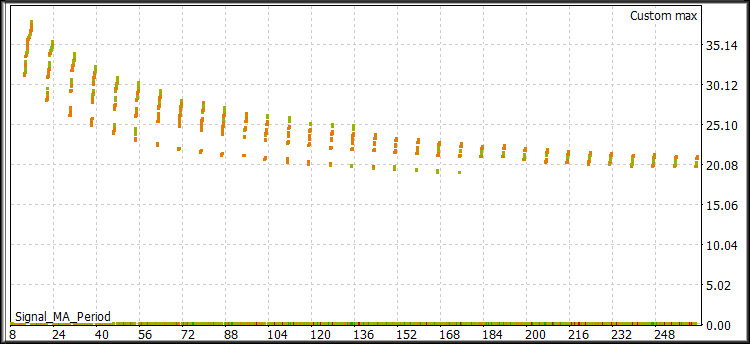• "Period": 8;
• "Timeframe": 2 Minutes (两分钟);
• "MA methods": Linear weighted (线性加权);
• "Calculation price": High price (最高价).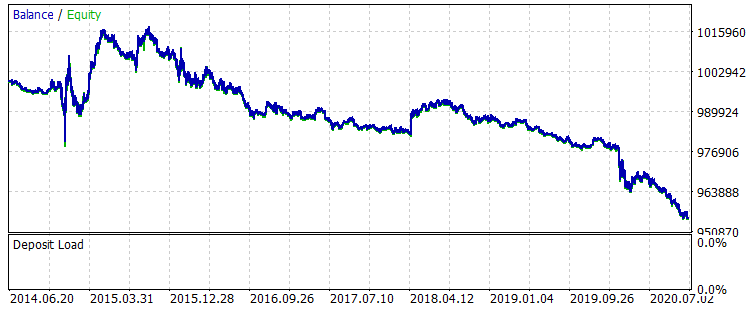• "Calculate predictors" — 设为 "true";
• "Save predictors" — 设为 "true";
• "Volume type in indicators" — 设置交易量类型: 报价点数(ticks)或是真实交易量;
• "Show predictor indicators on a chart" — 使用 "false";
• "Commission and spread in points to calculate target" — 设为 50.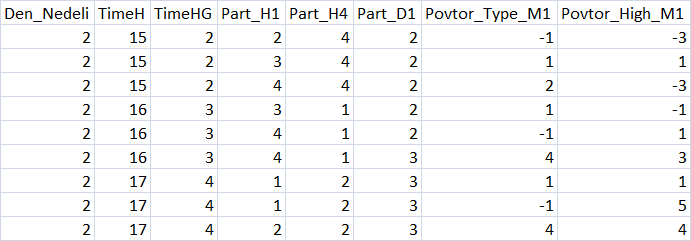### 拆分样本

• train.csv — 用于训练的样本
• test.csv — 用于控制训练结果的样本，也用于停止训练
• exam.csv — 评估训练结果的样本

```2408    Auxiliary
2409    Auxiliary
2410    Label
2411    Auxiliary
2412    Auxiliary
```

CatBoost 参数

CatBoost 有各种影响训练结果的参数和设置；它们都在这里解释。我将在这里提到对模型训练结果有更大影响的主要参数（以及它们的键值，如果有的话），这些参数可以在CB_Bat脚本中配置：

• "Project directory" — 指定“Setup”示例所在目录的路径；
• "CatBoost exe file name" — 我使用了catboost-0.24.1.exe版本；您应该指定您使用的版本；
• "Boosting type (Model boosting scheme)" — 选择两个提升选项：
• Ordered — 小数据集的质量更好，但速度可能较慢。
• Plain — 经典的梯度提升方案。
• "Tree depth" (depth) — 对称决策树的深度，开发者推荐值在6到10之间；
• "Maximum iterations (trees)" — 解决机器学习问题时可以建立的最大树数；学习后的树数可以较少。如果测试或验证样本中没有出现模型改进，则迭代次数应与学习率参数的变化成比例地改变；
• "Learning rate" — 梯度步长，即建立每个后续决策树时的泛化准则。值越低，训练越慢，也越精确，但是这将花费更长的时间，并且将产生更多的迭代，所以不要忘记更改“Maximum number of iterations (trees)”；
• "Method for automated calculation of target class weights" (class-weights) — 此参数允许通过每个类中的多个示例来改进对不平衡样本的训练。三种不同的平衡方法：
• None — 所有类权重都设置为1
• Balanced — 基于总权重的类权重
• SqrtBalanced — 基于每个类中对象总数的类权重
• "Method for selecting object weights" (bootstrap-type) — 该参数负责在搜索预测因子以构建新树时如何计算对象。以下选项可用：
• Bayesian;
• Bernoulli;
• MVS;
• No;
• "Range of random weights for object selection" (bagging-temperature) — 当选择贝叶斯方法作为预测搜索的计算对象时，使用该方法。这个参数在为树选择预测值时会增加随机性，这有助于避免过度拟合和寻找模式。参数可以取从零到无穷大的值。
• "Frequency to sample weights and objects when building trees" (sampling-frequency) — 允许在构建树时更改预测因子重新评估的频率。支持的值：
• PerTree — 在构建每棵新树之前
• PerTreeLevel — 在选择树的每个新分支之前
• "Random subspace method (rsm) — 每个训练步骤1分析的预测因子百分比，1=100%。参数的减小加快了训练过程，增加了一些随机性，但增加了最终模型中的迭代次数（树）；
• "L2 regularization" (l2-leaf-reg) — 从理论上讲，该参数可以减少过拟合，影响模型的质量；
• "The random seed used for training" (random-seed) — 通常是训练开始时随机权系数的发生器。根据我的经验，这个参数显著影响模型训练；
• "The amount of randomness to score the tree structure (random-strength)" — 此参数在创建树时会影响狭缝分数，对其进行优化以提高模型质量；
• "Number of gradient steps to select a value from the list" (leaf-estimation-iterations) — 当树已经建好的时候，叶子就会被计算在内。它们可以向前数几个梯度步-这个参数影响训练质量和速度；
• "The quantization mode for numerical features" (feature-border-type) — 此参数负责对样本对象执行不同的量化算法。参数对模型的可训练性影响很大。支持的值：
• Median,
• Uniform,
• UniformAndQuantiles,
• MaxLogSum,
• MinEntropy,
• GreedyLogSum,
• "The number of splits for numerical features" (border-count) — 此参数负责每个预测因子的整个值范围的分割数。分裂的数量通常较少。参数越大，分割越窄 -> 示例百分比越低。对学习种子和学习质量有显著影响；
• "Save borders to a file" (output-borders-file)  — 量化边界可以保存到一个文件中，以便在后续的训练中进行进一步的分析。它会影响学习速度，因为每次创建模型时都会保存数据；
• "Error score metrics for learning correction" (loss-function) — 训练模型时用来评估错误分数的函数。我没有注意到对结果的重大影响。有两种选择：
• Logloss;
• CrossEntropy;
• "The number trees without improvements to stop training" (od-wait) — 如果训练很快停止，试着增加等待的次数。学习速度变化时也要改变参数：速度越低，完成训练前等待改进的时间越长；
• "Error score metric function to training" (eval-metric)  — 允许从列表中选择一个度量，根据该度量树将被截断并停止训练。支持的量度：
• Logloss;
• CrossEntropy;
• Precision;
• Recall;
• F1;
• BalancedAccuracy;
• BalancedErrorRate;
• MCC;
• Accuracy;
• CtrFactor;
• NormalizedGini;
• BrierScore;
• HingeLoss;
• HammingLoss;
• ZeroOneLoss;
• Kappa;
• WKappa;
• LogLikelihoodOfPrediction;
• "Sample object" — 允许选择模型参数进行训练。选项:
• No
• Random-seed — 用于训练的值
• Random-strength — 评估树结构的随机性数量
• Border-count — 切分的次数
• l2-Leaf-reg — L2 规范化
• Bagging-temperature — 用于选择对象的随机权重范围
• Leaf_estimation_iterations — 从列表中选择值的渐变步数
• "Initial variable value" — 设置训练开始的位置
• "End variable value" — 设定训练结束的位置
• "Step" — 数值变化步长
• "Classification result presentation type"(prediction-type) — 如何编写模型响应-不影响训练，在训练后将模型应用于样本时使用：
• Probability
• Class
• RawFormulaVal
• Exponent
• LogProbability
• "The number of trees in the model, 0 - all" — 模型中用于分类的树的数量，允许在模型应用于样本时评估分类质量的变化
• "Model analysis method" (fstr-type) —各种模型分析方法可以评估某一模型的预测显著性。 请分享你对它们的看法。支持的选项:
• PredictionValuesChange — 对象值更改时预测如何更改
• LossFunctionChange — 排除对象时预测如何更改
• InternalFeatureImportance
• Interaction
• InternalInteraction
• ShapValues

### 学习策略

1. 基本设置是负责模型中树的深度和数量的参数，以及用于开始训练过程的训练速率、类权重和其他设置。不会搜索这些参数；在大多数情况下，脚本生成的默认设置就足够了。
2. 搜索最优分割参数 - CatBoost 对预测因子表进行预处理，沿网格边界搜索值范围，因此我们需要找到一个训练效果更好的网格。迭代范围为8-512的所有网格类型是有意义的；我在每个值上使用步长增量：8、16、32等等。
3. 再次配置脚本，指定找到的预测因子量化网格，然后我们可以继续处理进一步的参数。通常我只在1-1000范围内使用“Seed”。

• _00_Dir_All.txt - 辅助文件
• _01_Train_All.txt - 训练设置
• _02_Rezultat_Exam.txt - 按检查样本模型记录分类的设置
• _02_Rezultat_test.txt - 按测试样本模型记录分类的设置
• _02_Rezultat_Train.txt - 按学习样本模型记录分类的设置
• _03_Metrik_Exam.txt - 用于记录检查样本模型的每个树的度量的设置
• _03_Metrik_Test.txt - 用于记录测试样本模型的每个树的度量的设置
• _03_Metrik_Train.txt - 用于记录训练样本模型的每个树的度量的设置
• _04_Analiz_Exam.txt - 用于记录检查样本模型的预测值重要性评估的设置
• _04_Analiz_Test.txt - 用于记录测试样本模型的预测重要性评估的设置
• _04_Analiz_Train.txt - 用于记录训练样本模型的预测值重要性评估的设置

### 模型的训练

• _00_Dir_All.bat - 辅助文件
• _01_Train_All.bat - 训练的设置
• _02_Rezultat_Exam.bat - 按检查样本模型记录分类的设置
• _02_Rezultat_test.bat - 按测试样本模型记录分类的设置
• _02_Rezultat_Train.bat — 按学习样本模型记录分类的设置
• _03_Metrik_Exam.bat — 用于记录检查样本模型的每个树的度量的设置
• _03_Metrik_Test.bat — 用于记录测试样本模型的每个树的度量的设置
• _03_Metrik_Train.bat — 用于记录训练样本模型的每个树的度量的设置
• _04_Analiz_Exam.bat — 用于记录检查样本模型的预测值重要性评估的设置
• _04_Analiz_Test.bat — 用于记录测试样本模型的预测重要性评估的设置
• _04_Analiz_Train.bat — 用于记录训练样本模型的预测值重要性评估的设置
• catboost-0.24.1.exe — 用于训练 CatBoost 模型的可执行文件
• train.csv — 用于训练的样本
• test.csv — 用于控制训练结果的样本，也用于停止训练
• exam.csv — 用于评估结果的样本
• Test_CB_Setup_0_000000000//包含有关用于训练的示例列的信息的文件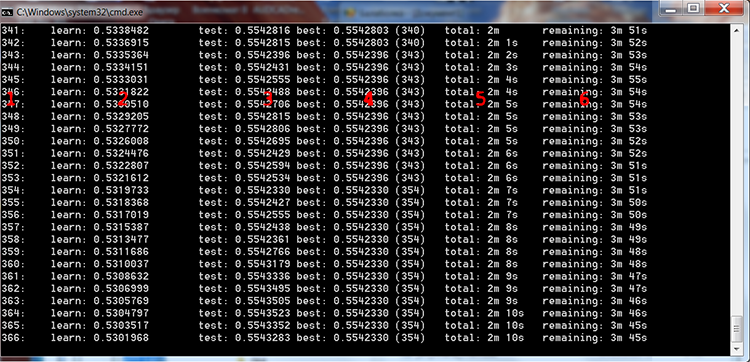1. 树的数目，等于迭代的次数
2. 在训练样本上计算所选损失函数的结果
3. 对控制样本计算所选损失函数的结果
4. 对控制样本计算所选损失函数的最佳结果
5. 模型训练开始后经过的实际时间
6. 如果训练设置指定的所有树，则训练结束前的估计剩余时间

```FOR %%a IN (*.) DO (
catboost-0.24.1.exe fit  --learn-set train.csv   --test-set test.csv     --column-description %%a        --has-header    --delimiter ;   --model-format CatboostBinary,CPP       --train-dir ..\Rezultat\RS_16\result_4_%%a      --depth 6       --iterations 1000       --nan-mode Forbidden    --learning-rate 0.03    --rsm 1         --fold-permutation-block 1      --boosting-type Plain   --l2-leaf-reg 6         --loss-function Logloss         --use-best-model        --eval-metric Logloss   --custom-metric Logloss         --od-type Iter  --od-wait 100   --random-seed 0         --random-strength 1     --auto-class-weights SqrtBalanced       --sampling-frequency PerTreeLevel       --border-count 16       --feature-border-type MinEntropy        --output-borders-file quant_4_00016.csv         --bootstrap-type Bayesian       --bagging-temperature 1         --leaf-estimation-method Newton         --leaf-estimation-iterations 10
catboost-0.24.1.exe fit  --learn-set train.csv   --test-set test.csv     --column-description %%a        --has-header    --delimiter ;   --model-format CatboostBinary,CPP       --train-dir ..\Rezultat\RS_32\result_4_%%a      --depth 6       --iterations 1000       --nan-mode Forbidden    --learning-rate 0.03    --rsm 1         --fold-permutation-block 1      --boosting-type Plain   --l2-leaf-reg 6         --loss-function Logloss         --use-best-model        --eval-metric Logloss   --custom-metric Logloss         --od-type Iter  --od-wait 100   --random-seed 0         --random-strength 1     --auto-class-weights SqrtBalanced       --sampling-frequency PerTreeLevel       --border-count 32       --feature-border-type MinEntropy        --output-borders-file quant_4_00032.csv         --bootstrap-type Bayesian       --bagging-temperature 1         --leaf-estimation-method Newton         --leaf-estimation-iterations 10
catboost-0.24.1.exe fit  --learn-set train.csv   --test-set test.csv     --column-description %%a        --has-header    --delimiter ;   --model-format CatboostBinary,CPP       --train-dir ..\Rezultat\RS_48\result_4_%%a      --depth 6       --iterations 1000       --nan-mode Forbidden    --learning-rate 0.03    --rsm 1         --fold-permutation-block 1      --boosting-type Plain   --l2-leaf-reg 6         --loss-function Logloss         --use-best-model        --eval-metric Logloss   --custom-metric Logloss         --od-type Iter  --od-wait 100   --random-seed 0         --random-strength 1     --auto-class-weights SqrtBalanced       --sampling-frequency PerTreeLevel       --border-count 48       --feature-border-type MinEntropy        --output-borders-file quant_4_00048.csv         --bootstrap-type Bayesian       --bagging-temperature 1         --leaf-estimation-method Newton         --leaf-estimation-iterations 10
catboost-0.24.1.exe fit  --learn-set train.csv   --test-set test.csv     --column-description %%a        --has-header    --delimiter ;   --model-format CatboostBinary,CPP       --train-dir ..\Rezultat\RS_64\result_4_%%a      --depth 6       --iterations 1000       --nan-mode Forbidden    --learning-rate 0.03    --rsm 1         --fold-permutation-block 1      --boosting-type Plain   --l2-leaf-reg 6         --loss-function Logloss         --use-best-model        --eval-metric Logloss   --custom-metric Logloss         --od-type Iter  --od-wait 100   --random-seed 0         --random-strength 1     --auto-class-weights SqrtBalanced       --sampling-frequency PerTreeLevel       --border-count 64       --feature-border-type MinEntropy        --output-borders-file quant_4_00064.csv         --bootstrap-type Bayesian       --bagging-temperature 1         --leaf-estimation-method Newton         --leaf-estimation-iterations 10
catboost-0.24.1.exe fit  --learn-set train.csv   --test-set test.csv     --column-description %%a        --has-header    --delimiter ;   --model-format CatboostBinary,CPP       --train-dir ..\Rezultat\RS_80\result_4_%%a      --depth 6       --iterations 1000       --nan-mode Forbidden    --learning-rate 0.03    --rsm 1         --fold-permutation-block 1      --boosting-type Plain   --l2-leaf-reg 6         --loss-function Logloss         --use-best-model        --eval-metric Logloss   --custom-metric Logloss         --od-type Iter  --od-wait 100   --random-seed 0         --random-strength 1     --auto-class-weights SqrtBalanced       --sampling-frequency PerTreeLevel       --border-count 80       --feature-border-type MinEntropy        --output-borders-file quant_4_00080.csv         --bootstrap-type Bayesian       --bagging-temperature 1         --leaf-estimation-method Newton         --leaf-estimation-iterations 10
catboost-0.24.1.exe fit  --learn-set train.csv   --test-set test.csv     --column-description %%a        --has-header    --delimiter ;   --model-format CatboostBinary,CPP       --train-dir ..\Rezultat\RS_96\result_4_%%a      --depth 6       --iterations 1000       --nan-mode Forbidden    --learning-rate 0.03    --rsm 1         --fold-permutation-block 1      --boosting-type Plain   --l2-leaf-reg 6         --loss-function Logloss         --use-best-model        --eval-metric Logloss   --custom-metric Logloss         --od-type Iter  --od-wait 100   --random-seed 0         --random-strength 1     --auto-class-weights SqrtBalanced       --sampling-frequency PerTreeLevel       --border-count 96       --feature-border-type MinEntropy        --output-borders-file quant_4_00096.csv         --bootstrap-type Bayesian       --bagging-temperature 1         --leaf-estimation-method Newton         --leaf-estimation-iterations 10
catboost-0.24.1.exe fit  --learn-set train.csv   --test-set test.csv     --column-description %%a        --has-header    --delimiter ;   --model-format CatboostBinary,CPP       --train-dir ..\Rezultat\RS_112\result_4_%%a     --depth 6       --iterations 1000       --nan-mode Forbidden    --learning-rate 0.03    --rsm 1         --fold-permutation-block 1      --boosting-type Plain   --l2-leaf-reg 6         --loss-function Logloss         --use-best-model        --eval-metric Logloss   --custom-metric Logloss         --od-type Iter  --od-wait 100   --random-seed 0         --random-strength 1     --auto-class-weights SqrtBalanced       --sampling-frequency PerTreeLevel       --border-count 112      --feature-border-type MinEntropy        --output-borders-file quant_4_00112.csv         --bootstrap-type Bayesian       --bagging-temperature 1         --leaf-estimation-method Newton         --leaf-estimation-iterations 10
catboost-0.24.1.exe fit  --learn-set train.csv   --test-set test.csv     --column-description %%a        --has-header    --delimiter ;   --model-format CatboostBinary,CPP       --train-dir ..\Rezultat\RS_128\result_4_%%a     --depth 6       --iterations 1000       --nan-mode Forbidden    --learning-rate 0.03    --rsm 1         --fold-permutation-block 1      --boosting-type Plain   --l2-leaf-reg 6         --loss-function Logloss         --use-best-model        --eval-metric Logloss   --custom-metric Logloss         --od-type Iter  --od-wait 100   --random-seed 0         --random-strength 1     --auto-class-weights SqrtBalanced       --sampling-frequency PerTreeLevel       --border-count 128      --feature-border-type MinEntropy        --output-borders-file quant_4_00128.csv         --bootstrap-type Bayesian       --bagging-temperature 1         --leaf-estimation-method Newton         --leaf-estimation-iterations 10
catboost-0.24.1.exe fit  --learn-set train.csv   --test-set test.csv     --column-description %%a        --has-header    --delimiter ;   --model-format CatboostBinary,CPP       --train-dir ..\Rezultat\RS_144\result_4_%%a     --depth 6       --iterations 1000       --nan-mode Forbidden    --learning-rate 0.03    --rsm 1         --fold-permutation-block 1      --boosting-type Plain   --l2-leaf-reg 6         --loss-function Logloss         --use-best-model        --eval-metric Logloss   --custom-metric Logloss         --od-type Iter  --od-wait 100   --random-seed 0         --random-strength 1     --auto-class-weights SqrtBalanced       --sampling-frequency PerTreeLevel       --border-count 144      --feature-border-type MinEntropy        --output-borders-file quant_4_00144.csv         --bootstrap-type Bayesian       --bagging-temperature 1         --leaf-estimation-method Newton         --leaf-estimation-iterations 10
catboost-0.24.1.exe fit  --learn-set train.csv   --test-set test.csv     --column-description %%a        --has-header    --delimiter ;   --model-format CatboostBinary,CPP       --train-dir ..\Rezultat\RS_160\result_4_%%a     --depth 6       --iterations 1000       --nan-mode Forbidden    --learning-rate 0.03    --rsm 1         --fold-permutation-block 1      --boosting-type Plain   --l2-leaf-reg 6         --loss-function Logloss         --use-best-model        --eval-metric Logloss   --custom-metric Logloss         --od-type Iter  --od-wait 100   --random-seed 0         --random-strength 1     --auto-class-weights SqrtBalanced       --sampling-frequency PerTreeLevel       --border-count 160      --feature-border-type MinEntropy        --output-borders-file quant_4_00160.csv         --bootstrap-type Bayesian       --bagging-temperature 1         --leaf-estimation-method Newton         --leaf-estimation-iterations 10
)
```

### 学习结果的评估解释

CB_Svod.csv 文件将包含各个样本中每个模型的指标以及财务结果。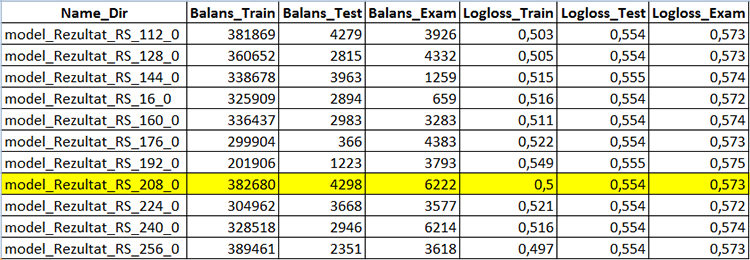```//If the CatBoost model is in an mqh file, comment the below line
//uint TreeDepth[];uint TreeSplits[];uint BorderCounts[];float Borders[];double LeafValues[];double Scale[];double Bias[];
#include "model_RS_208_0.mqh";                 // Model file
```

1. 配置预测因子的行为:

• "Calculate predictors" — 设为 "true";
• "Save predictors" — 设为 "false"
• "Volume type in indicators" — 选择您在训练中使用的交易量类型
• "Show predictor indicators on a chart" — 使用 "false"
• "Commission and spread in points to calculate target" — 使用之前的值，它不影响训练好的模型

2. 基本策略信号的MA指标参数：

• "Period": 8;
• "Timeframe": 2 Minutes (两分钟);
• "MA methods": Linear weighted (线性加权);
• "Calculation price": High price (最高价).

3. CatBoost模型应用参数：

• "Apply CatBoost model on data" — 设为 "true"
• "Threshold for classifying one by the model" — 保留为 0.5
• "Limit for classifying one by the model" — 保留为 1
• "Save model value to file" — 保留为 "false"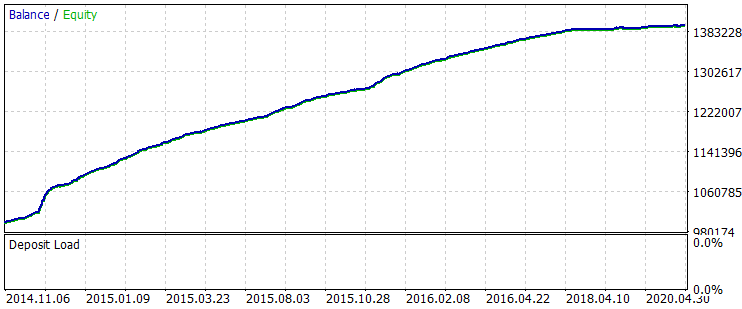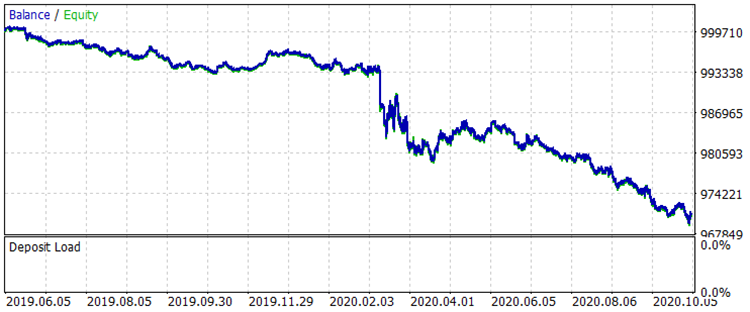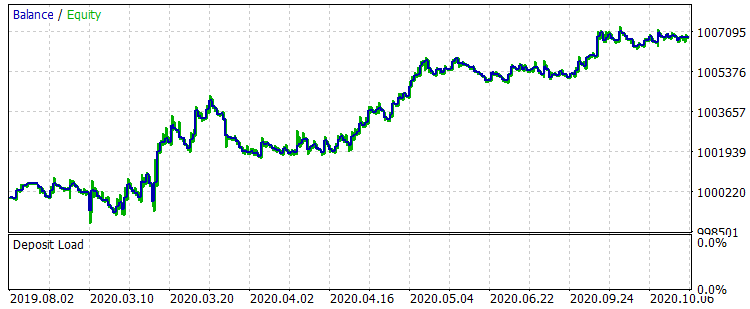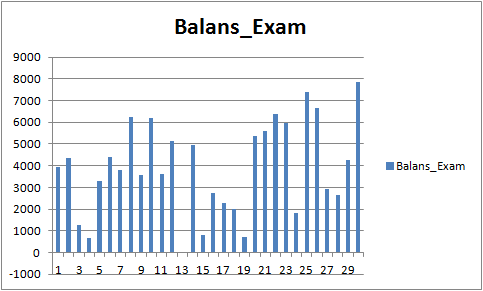### 预测因子分析

• Analiz_Train — 训练样本的预测因子重要性分析
• Analiz_Test — 检验（验证）样本的预测因子重要性分析
• Analiz_Exam — 测试（训练外）样本的预测因子重要性分析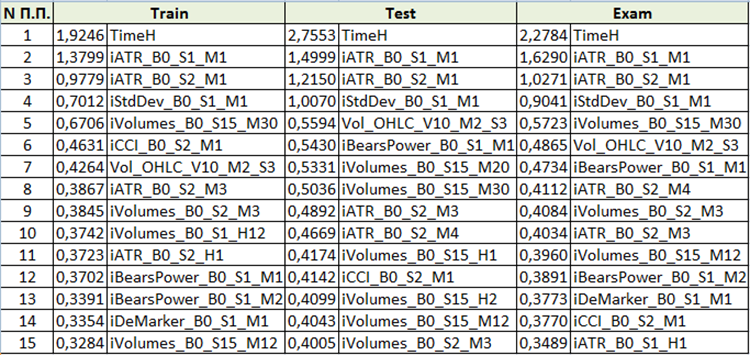### 结论

1. 机器学习方法的有效性，例如梯度提升，可以与无休止的参数迭代和手动创建额外的交易条件来提高策略性能相比。
2. 标准MetaTrader 5指标可以用于机器学习。
3. CatBoost — 是一个高质量的库，它有一个包装器，可以在不学习 Python 或 R 的情况下高效地使用梯度提升。

### 结论

MQL5.zip (111.56 KB)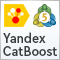基于暴力算法的 CatBoost 模型高级重采样与选择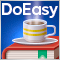DoEasy 函数库中的时间序列(第五十五部分)：指标集合类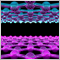市场及其全局模式中的物理学梯度提升（CatBoost）在交易系统开发中的应用. 初级的方法